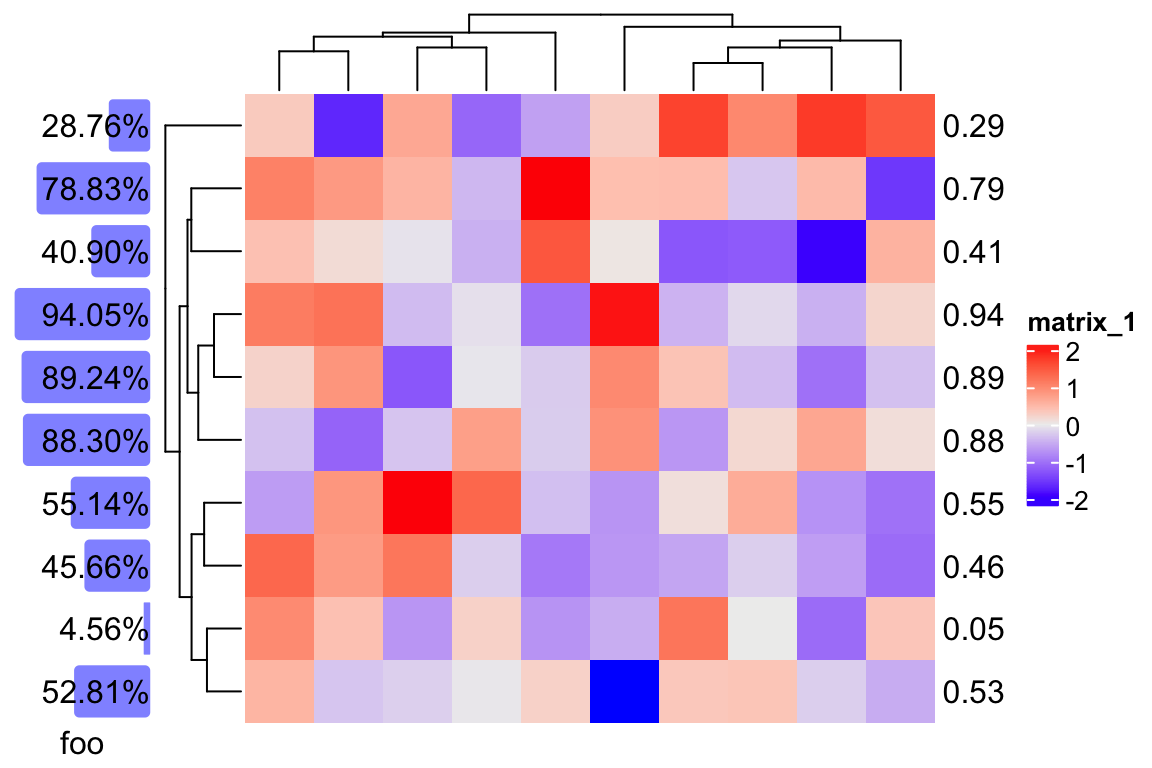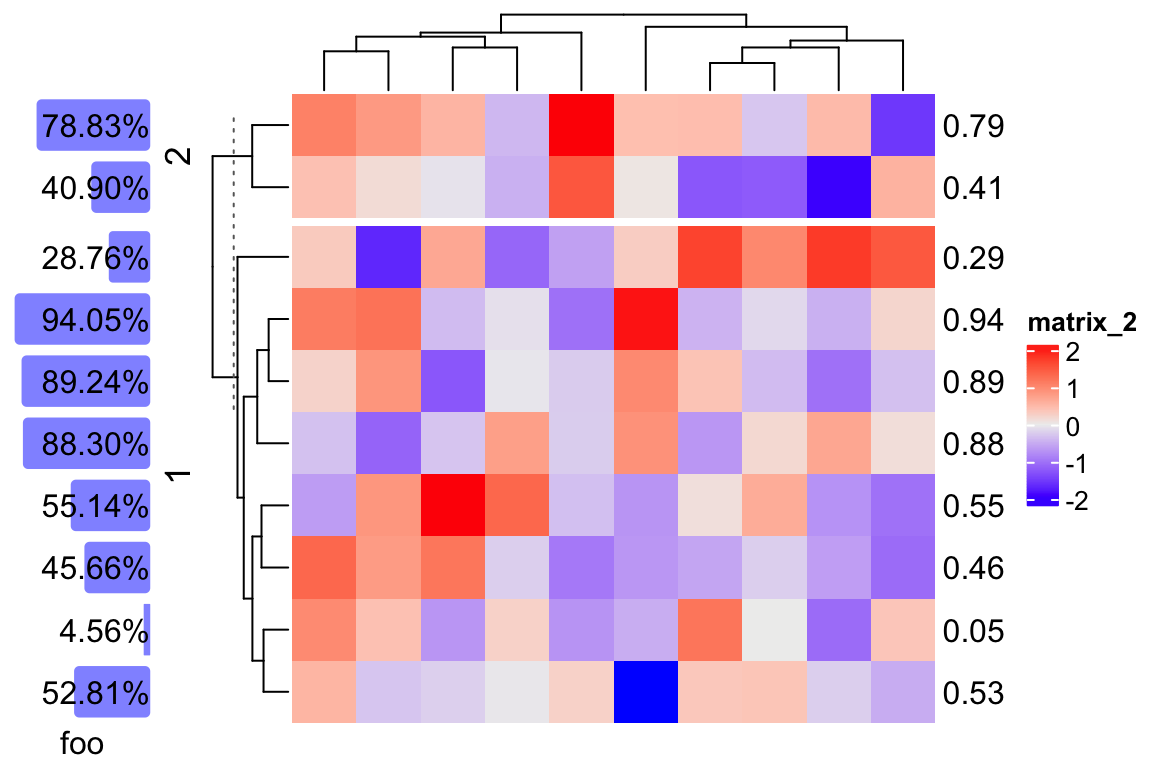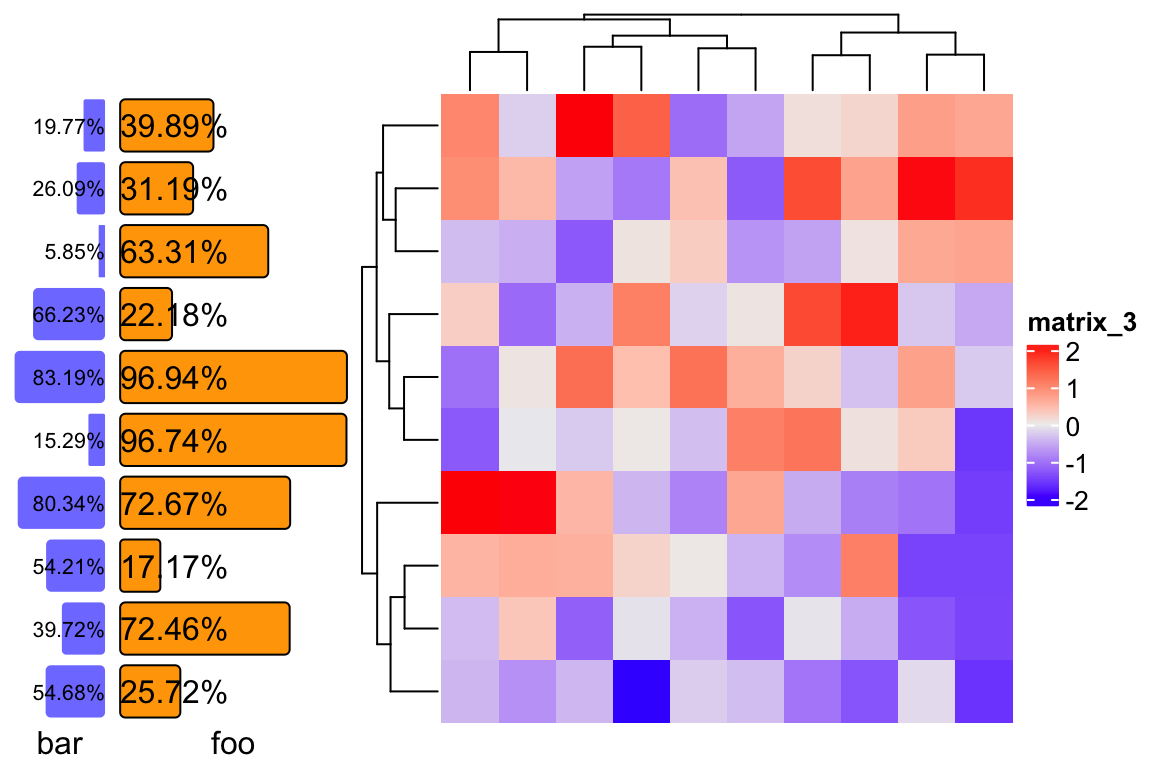# Create self-defined annotations

ComplexHeatmap already provides many annotation functions that draw various types of graphics, but still, users might want more new types. Here I demonstrate how to create new annotation graphics.

In the ComplexHeatmap book (https://jokergoo.github.io/ComplexHeatmap-reference/book/heatmap-annotations.html#implement-new-annotation-functions), I have already explained how to create new annotations with the function `AnnotationFunction()`. Here I demonstrate a simplified way to construct a new annotation type which also allows reordering, subsetting and splitting.

From version 2.9.3, `AnnotationFunction()` allows a new argument `cell_fun` which draws graphics in every single “annotation cell”. The value for `cell_fun` is a self-defind graphics function and the only argument is the “current index” of the corresponding heatmap rows or columns.

In the following example, I will create a new row annotation type which visualizes a list of percentage values by horizontal bars as well as the text of the percent values added on top of bars. I first give the function definition of `anno_pct()` which generate such annotation:

``````library(ComplexHeatmap)
anno_pct = function(x) {
max_x = max(x)
text = paste0(sprintf("%.2f", x*100), "%")
cell_fun_pct = function(i) {
pushViewport(viewport(xscale = c(0, max_x)))
grid.roundrect(x = unit(1, "npc"),
width = unit(x[i], "native"), height = unit(1, "npc") - unit(4, "pt"),
just = "right", gp = gpar(fill = "#0000FF80", col = NA))
grid.text(text[i], x = unit(1, "npc"), just = "right")
popViewport()
}

AnnotationFunction(
cell_fun = cell_fun_pct,
var_import = list(max_x, x, text),
which = "row",
width = max_text_width(text)*1.25
)
}``````

The code is simple. `cell_fun_pct()` draws a horizontal bar and a text for the ith row. Then `cell_fun_pct()` is sent to the constructor function to generate the new annotation.

We can test this new annotation function:

``````set.seed(123)
x = runif(10)
ha = rowAnnotation(foo = anno_pct(x), annotation_name_rot = 0)
m = matrix(rnorm(100), 10)
rownames(m) = round(x, 2)
ha + Heatmap(m)``````And if we split the heatmap rows:

``ha + Heatmap(m, row_km = 2)``We can make `anno_pct()` slightly more complex to allow more controls:

``````anno_pct = function(x, bg_gp = gpar(fill = "#8080FF", col = NA), labels_gp = gpar(),
align_to = "right", width = NULL) {

max_x = max(x)
text = paste0(sprintf("%.2f", x*100), "%")
cell_fun_pct = function(i) {
pushViewport(viewport(xscale = c(0, max_x)))
if(align_to == "right") {
grid.roundrect(x = unit(1, "npc"),
width = unit(x[i], "native"), height = unit(1, "npc") - unit(4, "pt"),
just = "right", gp = subset_gp(bg_gp, i))
grid.text(text[i], x = unit(1, "npc"), just = "right", gp = subset_gp(labels_gp, i))
} else {
grid.roundrect(x = unit(0, "npc"),
width = unit(x[i], "native"), height = unit(1, "npc") - unit(4, "pt"),
just = "left", gp = subset_gp(bg_gp, i))
grid.text(text[i], x = unit(0, "npc"), just = "left", gp = subset_gp(labels_gp, i))
}
popViewport()
}

if(is.null(width)) {
width = max_text_width(text, gp = labels_gp)*1.25
}
AnnotationFunction(
cell_fun = cell_fun_pct,
var_import = list(max_x, x, text, labels_gp, align_to, bg_gp),
which = "row",
width = width
)
}``````
``````ha1 = rowAnnotation(bar = anno_pct(runif(x), labels_gp = gpar(fontsize = 8)),
annotation_name_rot = 0)
ha2 = rowAnnotation(foo = anno_pct(runif(x), bg_gp = gpar(fill = "orange"),
align_to = "left", width = unit(3, "cm")), annotation_name_rot = 0)
m = matrix(rnorm(100), 10)
ha1 + ha2 + Heatmap(m)``````